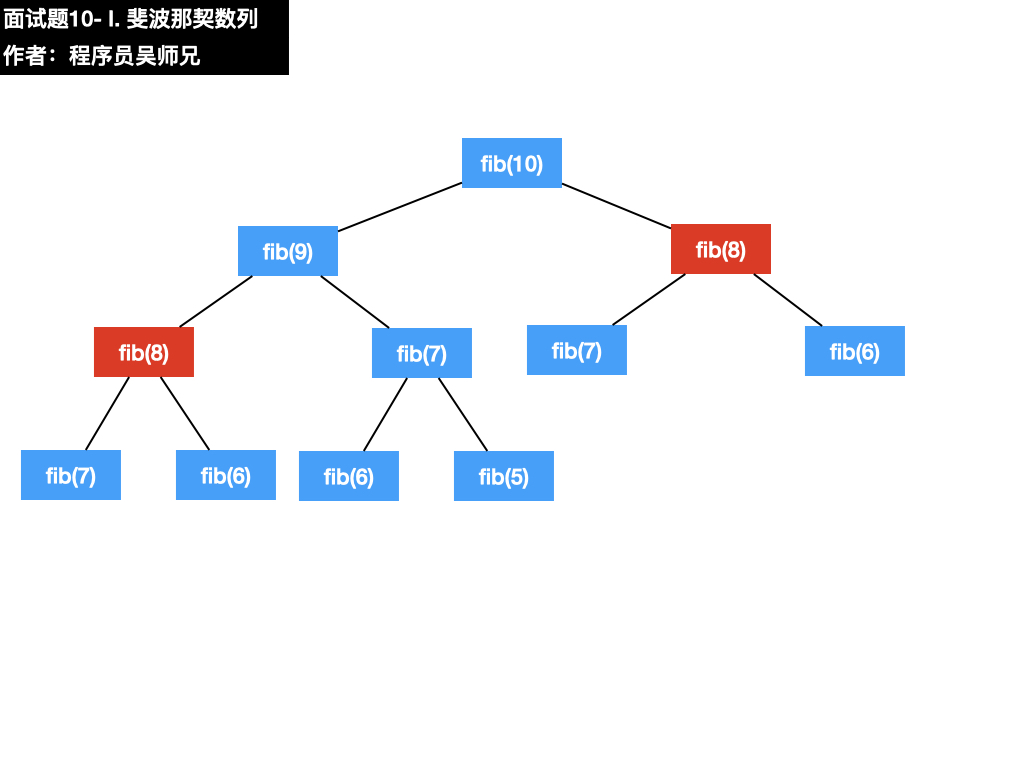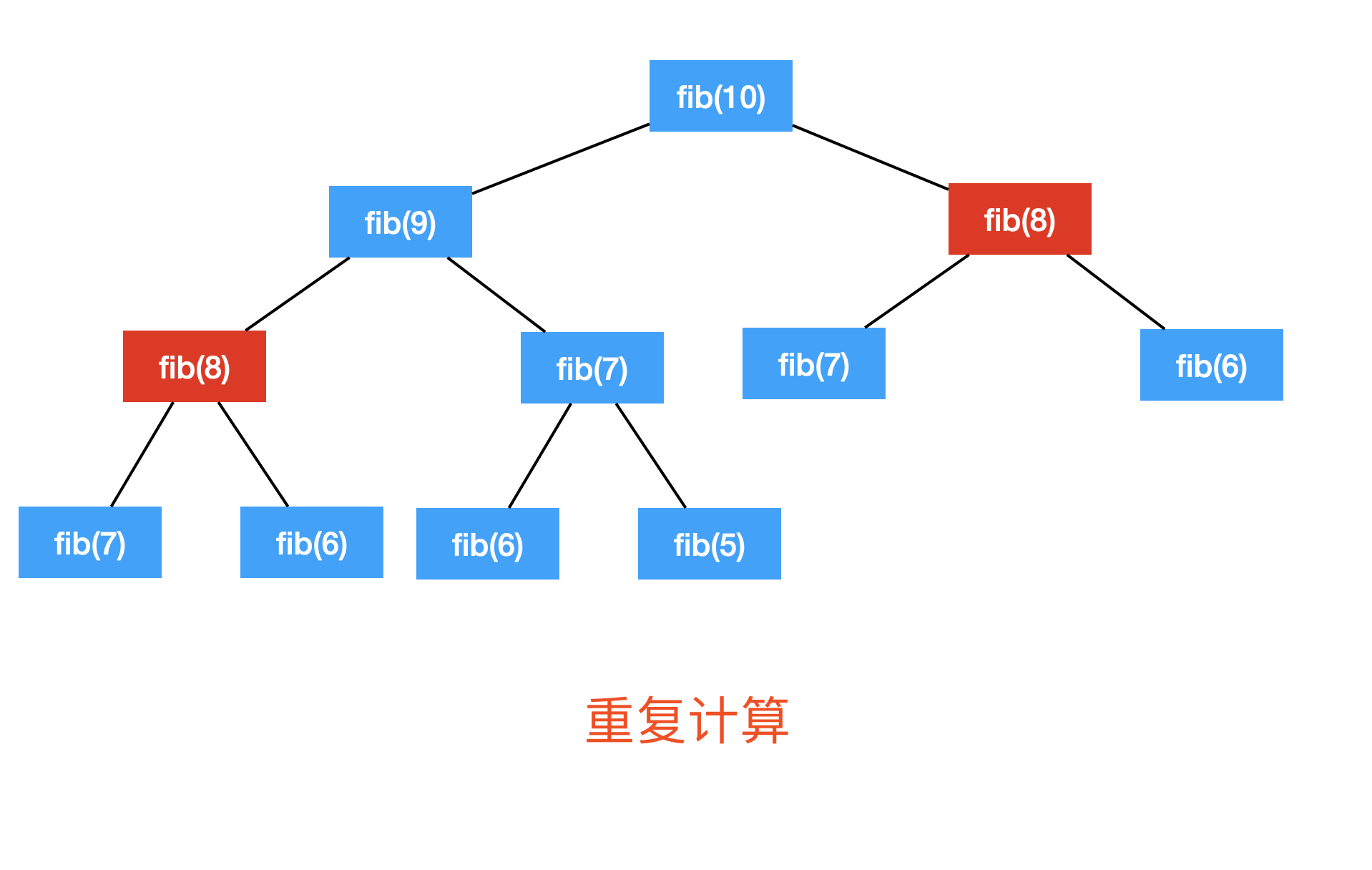https://www.algomooc.com/hi-offer

### 一、题目描述

F(0) = 0,   F(1) = 1
F(N) = F(N - 1) + F(N - 2), 其中 N > 1.


输入：n = 2



输入：n = 5



• 0 <= n <= 100

### 二、题目解析

class Solution {
public int fib(int n) {
if (n <= 0) return 0;
if (n == 1) return 1;
return (fib(n-1) + fib(n-2)) % 1000000007;
}
}• 模拟：模拟题目的运行。
• 规律：尝试总结出题目的一般规律和特点。
• 匹配：找到符合这些特点的数据结构与算法。
• 边界：考虑特殊情况。

#### 1、模拟### 三、动画理解

• 普通用户特权：5积分
• 会员用户特权：免费
• 算法训练营永久会员用户特权：免费推荐

### 四、参考代码

//访问网站，获取更多题解：https://www.algomooc.com
class Solution {
public int fib(int n) {
//边界判断
if(n == 0) return 0;
//用于存储第 0 到 n 个数对应的值
int[] dp = new int[n + 1];
//先定义好第一个数
dp = 0;
//再定义好第二个数
dp = 1;
//计算大于 0 和大于 1 的值
for(int i = 2; i <= n; i++){
//当遇到之前计算过的数时，将不再递归往下找，直接用记忆化结果
dp[i] = dp[i-1] + dp[i-2];
//题目要求进行取模处理
dp[i] %= 1000000007;
}
//返回结果
return dp[n];
}
}


• 递归
• 记忆化
• 备忘录
• 动态规划